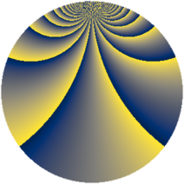# Properties

 Label 2280.2.faLevel $2280$ Weight $2$ Character orbit 2280.fa Rep. character $\chi_{2280}(557,\cdot)$ Character field $\Q(\zeta_{36})$ Dimension $5664$ Sturm bound $960$

# Related objects

## Defining parameters

 Level: $$N$$ $$=$$ $$2280 = 2^{3} \cdot 3 \cdot 5 \cdot 19$$ Weight: $$k$$ $$=$$ $$2$$ Character orbit: $$[\chi]$$ $$=$$ 2280.fa (of order $$36$$ and degree $$12$$) Character conductor: $$\operatorname{cond}(\chi)$$ $$=$$ $$2280$$ Character field: $$\Q(\zeta_{36})$$ Sturm bound: $$960$$

## Dimensions

The following table gives the dimensions of various subspaces of $$M_{2}(2280, [\chi])$$.

Total New Old
Modular forms 5856 5856 0
Cusp forms 5664 5664 0
Eisenstein series 192 192 0

## Trace form

 $$5664q - 24q^{6} - 24q^{7} + O(q^{10})$$ $$5664q - 24q^{6} - 24q^{7} - 24q^{10} - 6q^{12} - 24q^{15} - 60q^{16} - 24q^{18} - 48q^{25} - 72q^{28} - 6q^{30} - 48q^{31} + 12q^{33} - 24q^{36} - 24q^{40} + 24q^{42} - 24q^{46} + 72q^{48} - 24q^{52} + 72q^{55} - 24q^{57} - 192q^{58} - 12q^{60} - 108q^{63} + 48q^{66} - 24q^{70} + 36q^{72} - 48q^{73} + 24q^{76} - 18q^{78} - 48q^{81} + 264q^{82} - 12q^{87} + 180q^{88} - 12q^{90} + 48q^{96} - 48q^{97} + O(q^{100})$$

## Decomposition of $$S_{2}^{\mathrm{new}}(2280, [\chi])$$ into newform subspaces

The newforms in this space have not yet been added to the LMFDB.